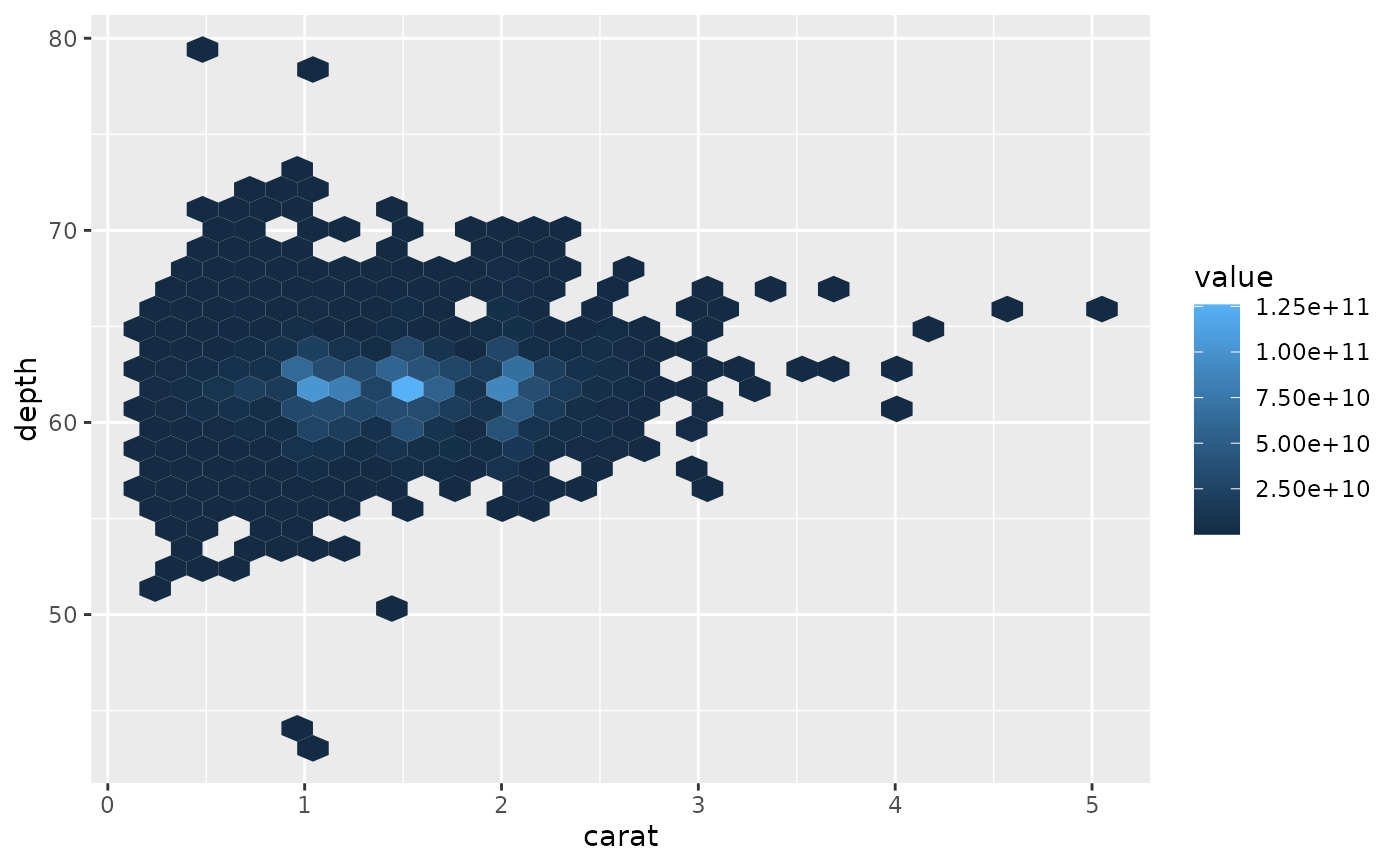stat_summary_2d() is a 2d variation of stat_summary(). stat_summary_hex() is a hexagonal variation of stat_summary_2d(). The data are divided into bins defined by x and y, and then the values of z in each cell is are summarised with fun.

## Usage

stat_summary_2d(
mapping = NULL,
data = NULL,
geom = "tile",
position = "identity",
...,
bins = 30,
binwidth = NULL,
drop = TRUE,
fun = "mean",
fun.args = list(),
na.rm = FALSE,
show.legend = NA,
inherit.aes = TRUE
)

stat_summary_hex(
mapping = NULL,
data = NULL,
geom = "hex",
position = "identity",
...,
bins = 30,
binwidth = NULL,
drop = TRUE,
fun = "mean",
fun.args = list(),
na.rm = FALSE,
show.legend = NA,
inherit.aes = TRUE
)

## Arguments

mapping

Set of aesthetic mappings created by aes(). If specified and inherit.aes = TRUE (the default), it is combined with the default mapping at the top level of the plot. You must supply mapping if there is no plot mapping.

data

The data to be displayed in this layer. There are three options:

If NULL, the default, the data is inherited from the plot data as specified in the call to ggplot().

A data.frame, or other object, will override the plot data. All objects will be fortified to produce a data frame. See fortify() for which variables will be created.

A function will be called with a single argument, the plot data. The return value must be a data.frame, and will be used as the layer data. A function can be created from a formula (e.g. ~ head(.x, 10)).

geom

The geometric object to use to display the data, either as a ggproto Geom subclass or as a string naming the geom stripped of the geom_ prefix (e.g. "point" rather than "geom_point")

position

Position adjustment, either as a string naming the adjustment (e.g. "jitter" to use position_jitter), or the result of a call to a position adjustment function. Use the latter if you need to change the settings of the adjustment.

...

Other arguments passed on to layer(). These are often aesthetics, used to set an aesthetic to a fixed value, like colour = "red" or size = 3. They may also be parameters to the paired geom/stat.

bins

numeric vector giving number of bins in both vertical and horizontal directions. Set to 30 by default.

binwidth

Numeric vector giving bin width in both vertical and horizontal directions. Overrides bins if both set.

drop

drop if the output of fun is NA.

fun

function for summary.

fun.args

A list of extra arguments to pass to fun

na.rm

If FALSE, the default, missing values are removed with a warning. If TRUE, missing values are silently removed.

show.legend

logical. Should this layer be included in the legends? NA, the default, includes if any aesthetics are mapped. FALSE never includes, and TRUE always includes. It can also be a named logical vector to finely select the aesthetics to display.

inherit.aes

If FALSE, overrides the default aesthetics, rather than combining with them. This is most useful for helper functions that define both data and aesthetics and shouldn't inherit behaviour from the default plot specification, e.g. borders().

## Aesthetics

• x: horizontal position

• y: vertical position

• z: value passed to the summary function

## Computed variables

These are calculated by the 'stat' part of layers and can be accessed with delayed evaluation.

• after_stat(x), after_stat(y)
Location.

• after_stat(value)
Value of summary statistic.

## Dropped variables

z

After binning, the z values of individual data points are no longer available.

stat_summary_hex() for hexagonal summarization. stat_bin2d() for the binning options.

## Examples

d <- ggplot(diamonds, aes(carat, depth, z = price))
d + stat_summary_2d()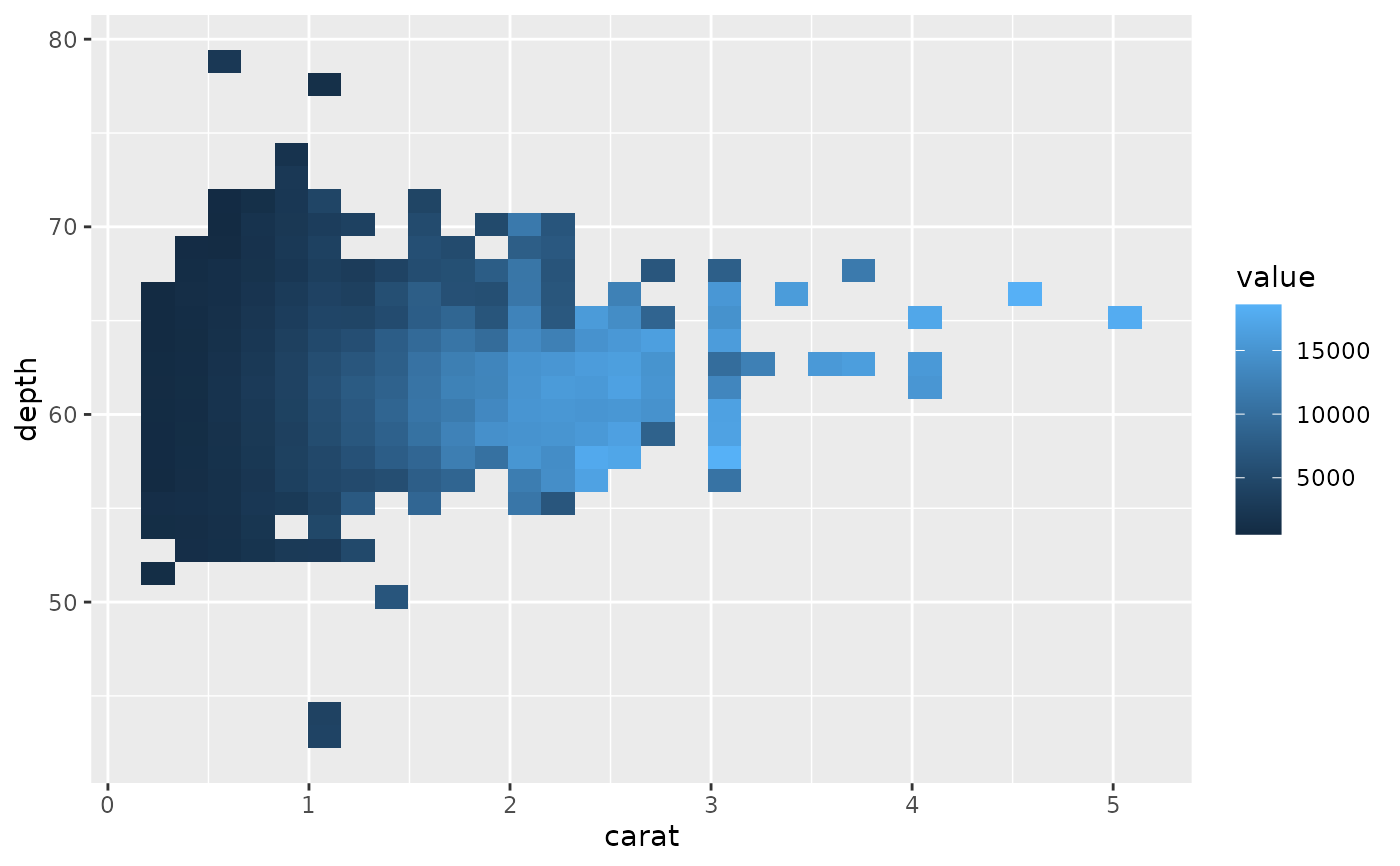# Specifying function
d + stat_summary_2d(fun = function(x) sum(x^2))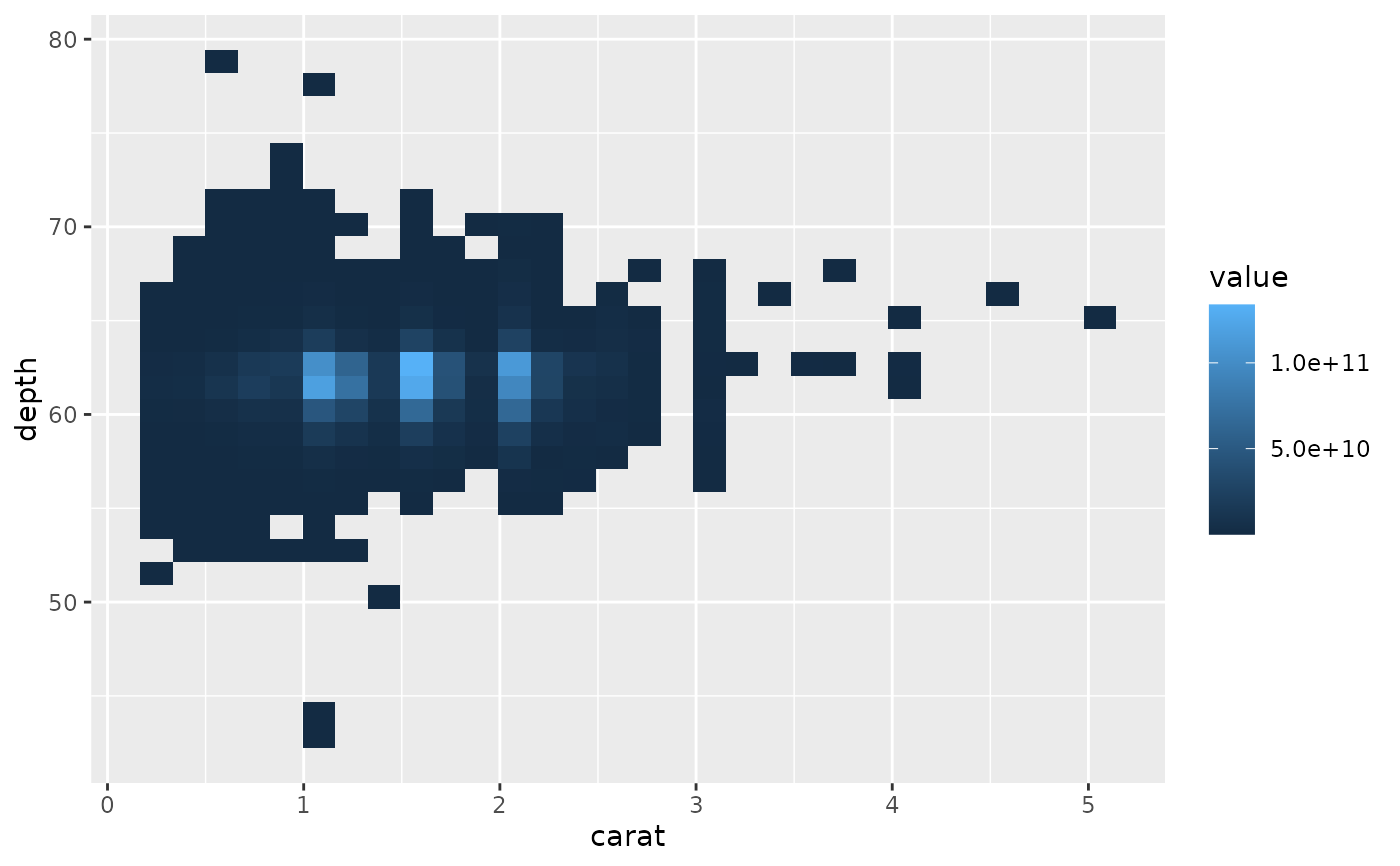d + stat_summary_2d(fun = ~ sum(.x^2))d + stat_summary_2d(fun = var)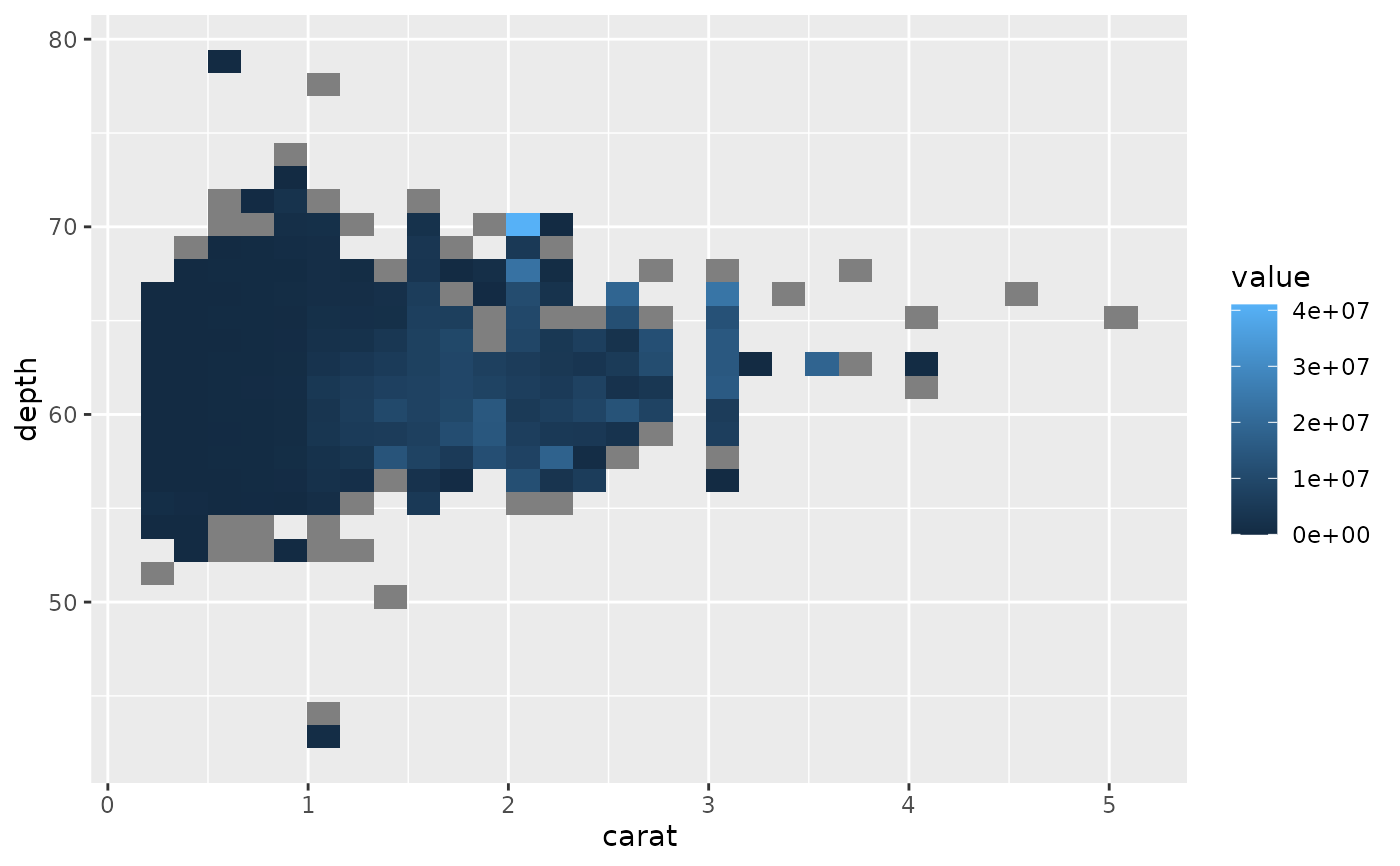d + stat_summary_2d(fun = "quantile", fun.args = list(probs = 0.1))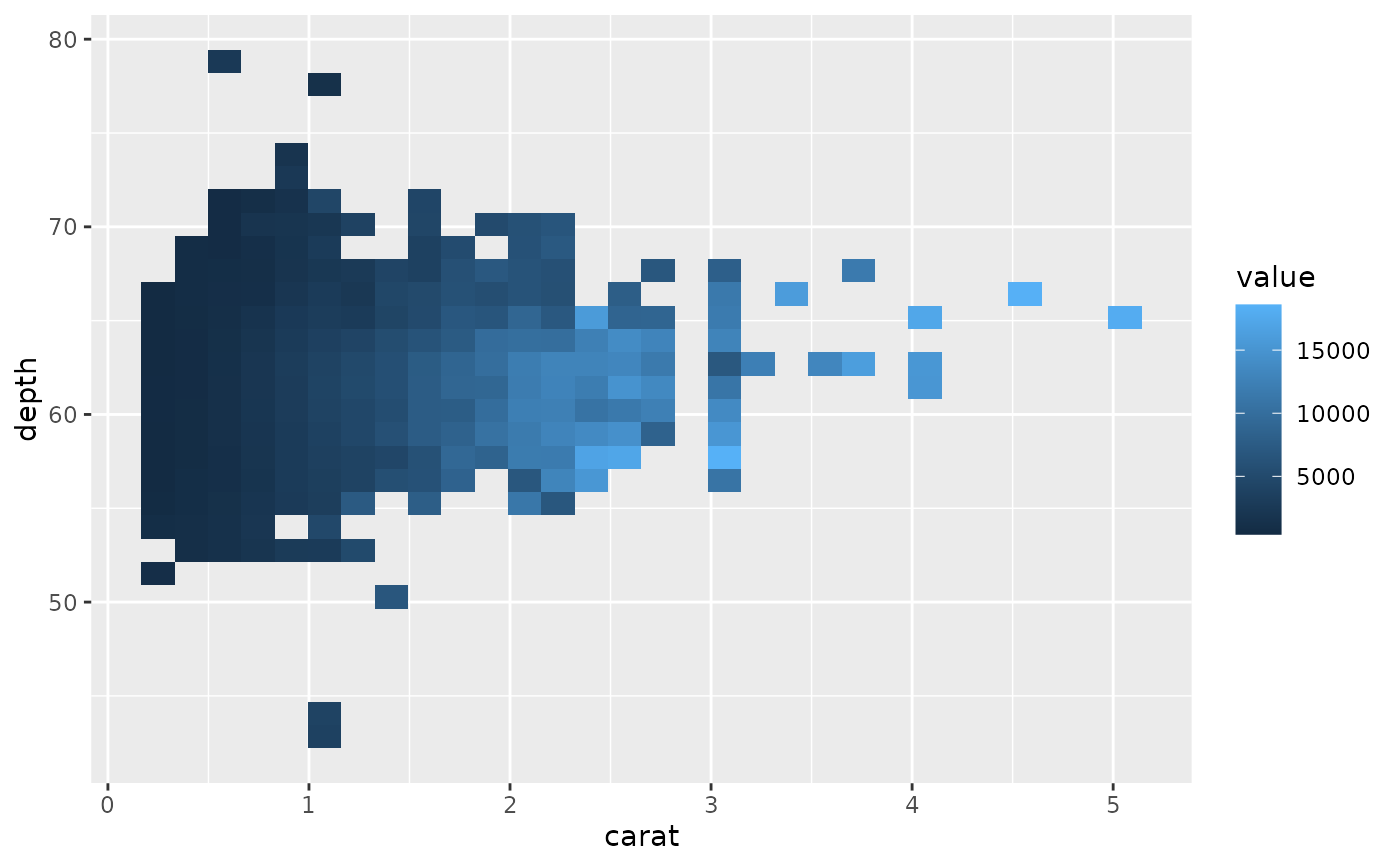if (requireNamespace("hexbin")) {
d + stat_summary_hex()
d + stat_summary_hex(fun = ~ sum(.x^2))
}